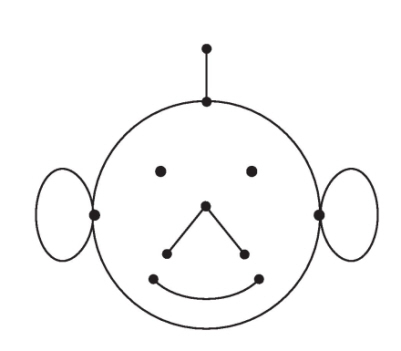# How does attaching a 1-cell to a path connected CW-complex affect the fundamental group?

If A is the path connected CW complex and X is the new CW complex made by attaching a single 1-cell, is it true that the fundamental group of X is the same as that of A?

I've tried to justify this via a proof I made but i'm not sure it's correct.

The 1-cell is isomorphic to the unit interval, so can treat X as $A\cup I$

Let $C=A \cup (0,\frac 34)$ and $D=(\frac 14 ,1)$ which are open subsets of X, $C \cup D=X$ and $C\cap D$ is path connected.

Then apply van Kampen to get $\pi_1(X,\frac 12)\cong\pi_1(C,\frac 12)*\pi_1(D,\frac 12)$ because N is trivial. But C deformation retracts to A and D is contractible so $\pi_1(X,\frac 12)\cong\pi_1(A,\frac 12)$.

Please correct my proof if it's wrong, currently I only know what is the equivalent of chapter 0 and 1 of Hatcher's book, so I don't know anything about homologys.

• If you attach a two cell, there should be two options. If the path where you're attaching the two cell is contractible, then nothing happens. If the path is nontrivial in the fundamental group, it now becomes trivial. – Michael Burr Mar 27 '16 at 17:08
• oops I meant 1-cell – Henry Mar 27 '16 at 17:13
• The proof is wrong because $C \cap D = A \cup (1/4, 3/4)$ has no reason to be path-connected. – user326188 Mar 27 '16 at 17:27
• Isn't $C \cap D = (1/4, 3/4)$? – Henry Mar 27 '16 at 17:31

## 3 Answers

Let $X$ be a path-connected topological space , and $f:(S^{n-1},p)\rightarrow (X,x_0)$ continuous and let $Y=X\cup _f e^n$ be the space obtained by attaching $e^n$ via $f$. Then

If $n\geq 3$ then the inclusion $X\hookrightarrow Y$ induces an isomorphism between $\pi _1 (X,x_0)\cong \pi _1 (Y,x_0)$.

If $n=2$ then $f$ defines an element $[f]\in \pi _1 (X,x_0)$ and $\pi _1 (Y,x_0)=\pi _1 (X,x_0)/[f]$.

If $n=1$ and $(X,x_0)$ is a correctly pointed topological space then $\pi _1 (Y,x_0)=\pi _1 (X,x_0)*\mathbb{Z}$.

Correctly pointed means there exists a neighbourhood $U$ of $x_0$ and a homotopy $H:V\times I\rightarrow V$, satisfying $H(x,0)=x$, $H(x,1)=x_0$ and $H(x_0,t)=x_0$ for all $t\in I$.

The theorem you should remember about attaching cells, proof uses Van Kampen (version from Félix, Tanré).

This is not true. If you consider $A$ to be a single point, then attaching a $1$-cell (in the only way possible) gives a circle, which is not simply connected.

• Ahh I see, but then how do I correct my proof (and which bit is wrong)? – Henry Mar 27 '16 at 17:32

It is often necessary to see the unit interval $I=[0,1]$ as a space with two base points $0,1$. This allows for attaching $1$-cells to $K^0$ to give cell complexes such as the following Fig 4.17 of Topology and Groupoids:You then need a Seifert-van Kampen Theorem for the fundamental groupoid $\pi_1(X,A)$ on a set $A$ of base points. The pushout theorem looks exactly like the usual theorem for $X=U \cup V$ but replaces

$\pi_1(X,a)$ with $U,V, W=U \cap V$ path connected and $a \in W$ by

$\pi_1(X,A)$ where $A$ meets each path component of $U,V,W$.

The proof, if done by verifying the universal property of a pushout, is not much different from the proof for the group case given by Crowell in 1959. Details are in this paper and in a different way in Topology and Groupoids (T&G). This idea was published in 1967.

See also Higgins' downloadable book Categories and Groupoids (1971). To interpret the theorem one needs to set up what can reasonably be called combinatorial groupoid theory, see T&G. This allows a treatment of constructions which include free groups and free products of groups, because of the "spatial extension to group theory" given by the objects of a groupoid. Note also that for $K$ a CW-complex, $\pi_1(K^1,K^0)$ is a free *groupoid**, so this idea needs developing. .

See also this mathoverflow discussion.

April 11, 2016 One aspect is that the groupoid $\mathcal I \cong \pi_1(I, \{0,1\})$ is a generator of the category of groupoids as $\mathbb Z$ is in the category of groups. Note also that the circle $S^1$ is obtained from $I$ by identifying $0,1$ in the category of spaces. And $\mathbb Z$ is obtained from $\mathcal I$ by identifying $0,1$ in the category of groupoids.

Further, $\mathcal I$ is an interval object in the category of groupoids, leading to a useful homotopy theory of groupoids.

There is still, and after almost 50 years, only one topology text which explains these facts.

• I am not sure how to interpret what seems to be a downgrading of a view that the unit interval naturally should have two base points. See also these comments of Grothendieck: mathoverflow.net/questions/220561/… .Answers of mine here and elsewhere are intended to help students to be aware of this alternative viewpoint in order to make their own judgement. – Ronnie Brown Apr 1 '16 at 20:40